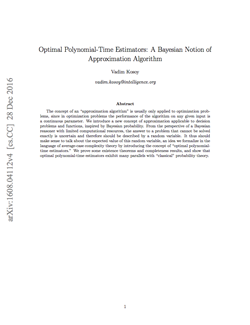# New paper: “Optimal polynomial-time estimators”

|   |  PapersMIRI Research Associate Vanessa Kosoy has developed a new framework for reasoning under logical uncertainty, “Optimal polynomial-time estimators: A Bayesian notion of approximation algorithm.” Abstract:

The concept of an “approximation algorithm” is usually only applied to optimization problems, since in optimization problems the performance of the algorithm on any given input is a continuous parameter. We introduce a new concept of approximation applicable to decision problems and functions, inspired by Bayesian probability. From the perspective of a Bayesian reasoner with limited computational resources, the answer to a problem that cannot be solved exactly is uncertain and therefore should be described by a random variable. It thus should make sense to talk about the expected value of this random variable, an idea we formalize in the language of average-case complexity theory by introducing the concept of “optimal polynomial-time estimators.” We prove some existence theorems and completeness results, and show that optimal polynomial-time estimators exhibit many parallels with “classical” probability theory.

Kosoy’s optimal estimators framework attempts to model general-purpose reasoning under deductive limitations from a different angle than Scott Garrabrant’s logical inductors framework, putting more focus on computational efficiency and tractability.

The framework has applications in game theory (Implementing CDT with Optimal Predictor Systems) and may prove useful for formalizing counterpossible conditionals in decision theory (Logical Counterfactuals for Random Algorithms, Stabilizing Logical Counterfactuals by Pseudorandomization),1 but seems particularly interesting for its strong parallels to classical probability theory and its synergy with concepts in complexity theory.

Optimal estimators allow us to assign probabilities and expectation values to quantities that are deterministic, but aren’t feasible to evaluate in polynomial time. This is context-dependent: rather than assigning a probability to an isolated question, an optimal estimator assigns probabilities simultaneously to an entire family of questions.

The resulting object turns out to be very natural in the language of average-case complexity theory, which makes optimal estimators interesting from the point of view of pure computational complexity, applications aside. In particular, the set of languages or functions that admit a certain type of optimal estimator is a natural distributional complexity class, and these classes relate in interesting ways to known complexity classes.

Optimal estimators can be thought of as a bridge between the computationally feasible and the computationally infeasible for idealized AI systems. It is often the case that we can find a mathematical object that answers a basic question in AI theory, but the object is computationally infeasible and so can’t model some key features of real-world AI systems and subsystems. Optimal estimators can be used in many cases to construct an optimal feasible approximation of the infeasible object, while retaining some nice properties analogous to those of the infeasible object.

To use estimators to build practical systems, we would first need to know how to build the right estimator, which may not be possible if there is no relevant uniform estimator, or if the relevant estimator is impractical itself.2 Since practical (uniform) optimal estimators are only known to mathematically exist in some very special cases, Kosoy considers estimators that require the existence of advice strings (roughly speaking: programs with infinitely long source code). This assumption implies that optimal estimators always exist, allowing us to use them as general-purpose theoretical tools for understanding the properties of computationally bounded agents.3

1. Kosoy’s framework has an interesting way of handling counterfactuals: one can condition on falsehoods, but only so long as one lacks the computational resources to determine that the conditioned statement is false.
2. For certain distributional estimation problems, uniform optimal estimators don’t exist (e.g., because there are several incomparable estimators such that we cannot simultaneously improve on all of them). In other cases, they may exist but we may not know how to construct them. In general, the conditions for the mathematical existence of uniform optimal estimators are an open problem and subject for future research.
3. Thanks to Vanessa Kosoy for providing most of the content for this post, and to Nate Soares and Matthew Graves for providing additional thoughts.